ECO256 --------------------------------------------------------------------------------------------------- Question: The difference between the definite and the indefinite integral is that,___ Answer: definite integral has limits Question: Using one of the rules of integration, an evaluation of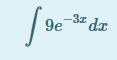is ___ Answer: \[-3e^{13x}+C\] Answer: -3e^{13x}+C Answer: -3e^(13x)+C Answer: -3e^ (13x) + C Question: If demand function is p=40-8q, the marginal revenue (MR) of the function will be ___ Answer: \[40-8q\] Answer: 40-8q Answer: 40 - 8q Question: When an equation is first partially differentiated w.r.t a variable, and then partially differentiated w.r.t another variable, this case is known as ___ Answer: Crossed partial derivative Question: When a constraint renders the 'substitution method' useless, ___ becomes effective Answer: Lagrenga multiplier method Question: An evaluation of the marginal expenditure of p=Q3+4Q+3 equals to ___ Answer: \[4Q^{3}+8Q+3\] Answer: 4Q^{3}+8Q+3 Answer: 4Q^(3)+8Q+3 Answer: 4Q^(3) + 8Q + 3 Question: The marginal propensity to consume (MPC) of the equation C=1000+0.88y is ___ Answer: \[0.88\] Answer: 0.88 Question: If the average propensity to save of a household is half, the average propensity to consume is ______ Answer: ½ Answer: Half Question: If MPC is 0.6, and consumption is 85, the consumption function 'C' is ___ Answer: \[0.6y+85\] Answer: 0.6y+85 Answer: 0.6y + 85 Question: The difference between the definite and the indefinite integral is that,___ Answer: definite integral has limits Question: Study the function F(x, y,ƛ) = 𝒇(x, y) + ƛ[k = h(x, y)] carefully: F(x, y,ƛ) is the _____ Answer: Lagrange function Question: 𝒇(x, y) in the function F(x, y,ƛ) = 𝒇(x, y) + ƛ[k = h(x, y)] is the ________ Answer: Objective function Question: In the function F(x, y,ƛ) = 𝒇(x, y) + ƛ[k = h(x, y)], ƛ[k = h(x, y)] is the ______ Answer: Constraint function Question: If g = 4w3 + 10wxy3 - y2 +x4 . With respect to ‘x’, the partial derivative of this function is______ Answer: 10wy3 + 4x3 Answer: 10wy^3 + 4x^3 Answer: 10wy^3+4x^3 Question: If g = 4w3 + 10wxy3 - y2 +x4 , the partial derivative of the function with respect to ‘w’ is ______ Answer: 12w + 10xy3 Answer: 12w + 10xy^3 Answer: 12w+10xy^3 Question: If g = 4w3 + 10wxy3 - y2 +x4 , with respect to ‘y’, the partial derivative is ______ Answer: 30wxy^2 – 2y Answer: 30wxy^2–2y Question: When the substitution method becomes useless as a result constraint, _______ becomes effective. Answer: Lagrange multiplier Question: In matrix operation, any matrix of 2 by 3 order means ______ Answer: 2 rows and 3 columns Answer: Two rows and three columns Answer: 2 rows, 3 columns Question: When the second derivative of any function equals zero, the _____ occurs Answer: inflection point Answer: point of inflection Question: The first among the rules of differentiation is the ______ Answer: Constant rule Answer: Constant Question: Use Lagrange multiplier to optimizesubject to x + y = 36. Therefore,The value of ‘y’ is_____ Answer: 15 Question: Use Lagrange multiplier to optimizesubject to x + y = 36. Therefore,The value of x in the equation is ____ Answer: 21 Question: Use Lagrange multiplier to optimizesubject to x + y = 36. Therefore,The estimated value of ƛ in the equation is______ Answer: 276 Question: Use Lagrange multiplier to optimizesubject to x + y = 36. Therefore,The value of q in the equation given is ______ Answer: 5,244 Question: The Marginal Revenue (MR) of the function Q = 46 – 2p is _____ Answer: 23 – Q Answer: 23–Q Question: Using 23–Q, if Q = 6, then MR is _______ Answer: #17 Answer: N17 Question: Total Revenue (TR) value of the function Q = 46 – 2p is ______ , if Q is 7.. Answer: #136.5 Answer: 136.5 Question: From the consumption function C = 2500 + 0.75Yd, the Marginal Propensity to Consume (MPC) is ______ Answer: 0.75 Question: The Marginal Propensity to Save (MPS) is ______ given the consumption function C = 2500 + 0.75Yd. Answer: 0.25 Question: The value of the consumer expenditure using the function C = 2500 + 0.75Yd is ______, if disposable income is #2500. Answer: #4375 Answer: #4,375 Answer: #4,357.00 Question: Given the Average Cost function, the Marginal Cost (MC) is ______ Answer: 5Q + 6 Answer: 5Q+6 Question: Using 5Q + 6, if Q is 4, MC value will be _____ Answer: #26 Answer: #26.00 Answer: Twenty-six naira Question: The value of Total Cost (TC) using the functionis ____,if Q equals 7. Answer: #220.50 Answer: #220.5 Answer: N220.5 Question: If MC = 70 + 90Q – 30Q2, and fixed cost is 100. The TC equation from the MC function is ______ Answer: 70Q + 45Q2 – 10Q3 + 100 Answer: 70Q + 45Q^2 – 10Q^3 + 100 Answer: 70Q+45Q^2–10Q^3+100 Question: The value of TC is X in absolute term when Q is 5. What is X? Answer: #325 Answer: #325.00 Question: Identify the generalized power function rule in differentiation if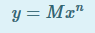Answer: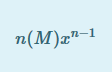Question: Solve the function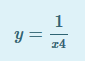using the rule of differentiation Answer: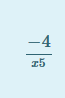Question: If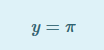, where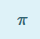is 3.142. Differentiate the function Answer: 0 Question: If the dependent variable is Y and the independent variable is x, find the derivative of the equation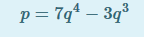Answer: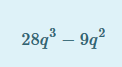Question: What is the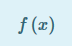of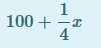Answer: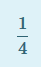Question: Differentiate the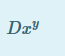of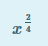Answer: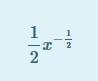Question: Use one of the rules of differentiation to solve the equation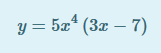Answer: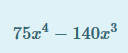Question: Given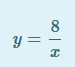, solve by finding its derivative Answer: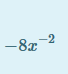Question: Find the derivative of the equation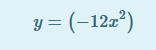Answer: -24x Question: The concept of Derivative is about ___ Answer: Rate of change Question: If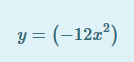differentiate it using one of the rules of Differentiation. Answer: -24x Question: Differentiation is a primitive function in calculus Answer: FALSE Question: What President Obama did by tracing his origin to Kenya can be likened to ___ in calculus Answer: Integration Question: The concept of Integration is about Answer: area under the curve Question: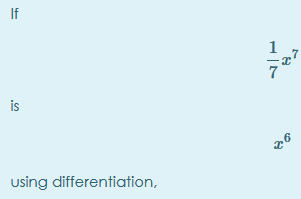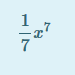is known as Answer: primitive function Question: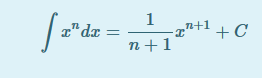in the rules integration is called Answer: Power function rule Question: Solve the derivative function x6, using the rule of integration Answer: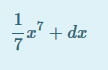Answer: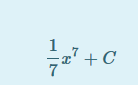Question: Identify the correct integration notation for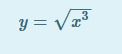Answer: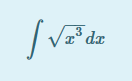Question: Use constant rule of integration, evaluate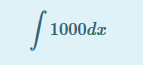Answer: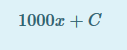Question: Compute the integral function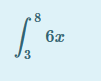Answer: 165 Question: Determine the under the curve of the function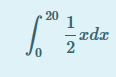Answer: 100 Question: If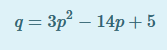, where p = 4, solve the equation to determine the functional form of the equation. Answer: Increasing Question: Solve to identify the nature of the function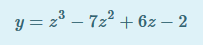, when z = 4 Answer: Decreasing Question: Solve equation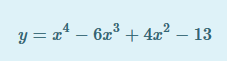when x = 4, and describe the state of the function. Answer: Stationary Question: When the first derivative of an economic model is zero or undefined, the model is therefore ___ Answer: Critical Question: In an economic equation where a single variable impact the endogenous variable is called ___ Answer: a parameter function Question: Find the partial derivative of the function,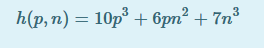w.r to p. Answer: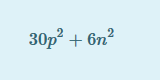Question: Determine the second derivative of function,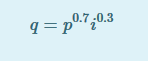w.r to i Answer: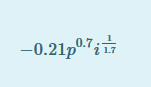Question: A column matrix is also known as ___ matrix Answer: m by 1
Question: The transpose of matrix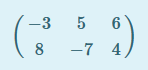is transformed to give matrix dimension ___ Answer: 3 by m Question: Find the product of the matrices ABAnswer: 65 Question: Find the Total Value of Sales (TVS), if Y is row vector of quantities of Biros, Rulers and Pencils respectively, and Z is a column vector of the corresponding prices of the goods.Answer: #52.29 Question: Cramer’s rule for matrix solution states that _____ Answer:Question: Using the answer to question 33, what is outcome of the matrix below?Answer: -10 Question: IfAnswer: -20